During the 2 hours journey to Stockholm from Bjursås, I found an interesting way of illustrating solutions to problems with a certain pattern. In this case, it was a recursion problem. The problem is as following (From Concrete Mathematics – A Foundation for Computer Science 2nd edition):

Problem

Solve the recurrence: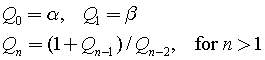Assume that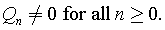Solution

When we look at several cases a pattern is going to be observed quite quickly.As you might see, the fifth and sixth terms turn out to be the initial conditions that were set in the beginning (see the first and second terms). Therefore, this recurrence will produce a repeating pattern.

The question we face at this stage is how the following can be described as a single expression.

My solution consists of five main steps:

1. Identify the common expression, i.e. the expression that contains all terms. In this case it’s the third expression. The expression should allow you to use it to express other cases by removing some terms. For example, the second term can be expressed in terms of the third if alpha in the numerator and beta in the denominator are not present. This is achieved by taking the terms that should not be present to the power of zero or multiply by zero, i.e,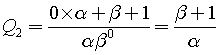2. Note down when each term in the common expression is present. If the term is present in specific case, write one, otherwise zero. For example, alpha in the numerator is present in cases 0, 3, 4. It’s also present in case 5, but we don’t take that into account because the pattern continues from the beginning. Now, do the same for all terms in the common expression so that you get an array of 1’s and 0’s for each term: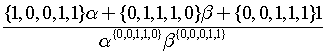.
You might wonder why there is a set of numbers in front of each term in the numerator and in the denominator, and that each term is raised to a set of 1’s and 0’s. Well, since we are generalizing each case in terms of the common expression, we can now say that if the case is zero, the set should be replaced by the first number in that set; when we deal with case one, we look at the second item, and so on. The reason why the terms in the numerator are multiplied by the set, while the ones in the denominator are raised to it, is because of the different operations. In the numerator, we are dealing with addition, and the only way to get rid of say alpha is by multiplication with zero. In the denominator, multiplying by zero will result an undefined expression. We don’t want undefined expressions, so we raise the terms in the denominator by zero or one. The effect is similar, we get 1 each time any term is raised to it, and it will therefore not affect the value of the fraction.
3. Observe the pattern in each set: By looking at this as a future computer scientist, a clear pattern was observed – the digits in each set represent coefficients of a number in radix 2. Interesting, maybe we can replace each set by a number instead. {1,0,0,1,1} represents 19, while {0,1,1,1,0} represents 14. This turns out to be a good idea, so we need to find a method that will return each digit in the binary number separately. My version of such function contains subtraction, floor, exponent only and can be found here in pdf.
4. Understand the new concept: The function I refereed to in step 3 looks as following: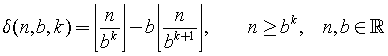It contains three parameters and some interesting properties.  Basically, the n is the number in base 10 that we want to convert to base 2, b is the base (in our case it’s 2) and k is the index of the digit in base 2 (so, since 14=1110, then when k=0 this function will return 0). There are both good news and bad news. Let’s start with the bad news. If we want to get a sequence like 0,1,1,1,0, it would imply the number 14. However, when we convert the number 14 back to this sequence, we get 1,1,1,0. In other words, no matter how many zeros we have on the left hand side, this will not affect the number in base 10. That is, 00011 has the value 11, but given 11 we do not automatically get the number of zeros that we started out with. Well, what’s the good news? It turns out that we can exploit a limitation of this function that will allow us to store these missing zeros. The limitation is that it does not support decimal numbers, since when the ratio between n/b^k gets small enough, the floor of it will always be equal to zero. This is a good thing as it allows us to generate additional zeros in the beginning.
5. Manipulate the expression to fit our needs: In step 4 we got some grasp of the function. Now, let’s put it into a context. Say we want to express {0,0,1,0,1,0,0,1}  using this function (let’s call it delta). If we would use this function, starting at k=0 to k=7 and setting n=41 and b=2, we would get {1,0,0,1,0,1,0,0} – the same thing but in reversed order. So, simply start the loop from k=7 to k=0 and the problem is solved, or start with k=0 to k=7 and change k=7-i, where i=0 to i=7.
6. Express the problem with the newly introduced concept: The “sets” in the expression in step 2 can be expressed with our new function. In the statements below, it is assumed that the loop starts at i=0 and ends at i=4.
• {1,0,0,1,1} = δ(19, 2, 4-i)
• {0,1,1,1,0} = δ(14, 2, 4-i)
• {0,0,1,1,1} = δ(7, 2, 4-i)
• {0,0,1,1,0} =δ(6, 2, 4-i)
• {0,0,0,1,1} =δ(3, 2, 4-i)

Note, δ(3, 2, 4-i) can be written as δ(3, 2, i) if the loop starts at i=4 and ends at i=0. Therefore,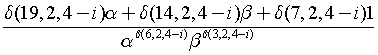or if we reverse the loop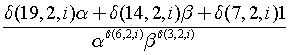Conclusion

Initially, this problem was all about to find a pattern in a recurrence. However, by trying to generalize the pattern in another way, new perspectives of looking at the pattern were found. Now, instead of stating the pattern with words, we can express it in a very condensed form. Moreover, the solution can be said to have several levels of abstractions. We have, in other words, modularized the problem into 1) a function that finds digit in base 2 – “the delta function” and 2) a common expression that uses the function to express the pattern. The group of 1 and 2 can be another abstraction level, i.e. we can now use this to solve other recurrences without going in to details of each individual part.

I think and hope that this way of looking at the problem will allow us to analyse different patterns more in details and find even more patterns that we have not thought about!